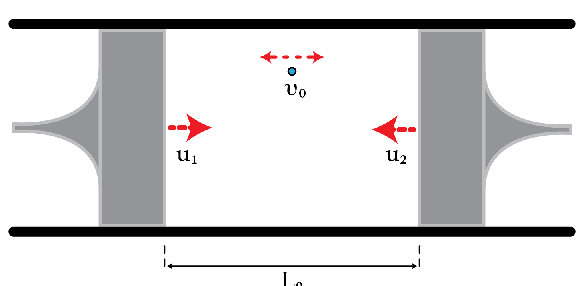# Compressing A Single-Particle GasConsider a particle moving between two pistons as shown in the figure. The distance between the pistons is reduced slowly (as slowly as you want), i.e., $v\gg u_{1}, u_{2}$ and the collisions are perfectly elastic. In this problem, we want to determine the equation of state relating the speed of the particle $v$ and the distance between the pistons $L$. Initially, the speed of the particle is $v_{0}=300~\text{m}/\text{s}$ and the distance between the pistons is $L_{0}=10~\text{cm}$. Find the speed of the particle in meters per second when the distance between the pistons becomes $\frac{L_{0}}{3}.$

×